1595082060

# Classification of Fruit Images Using Neural Networks (PyTorch)

Objective: Find a neural network model that achieves the highest accuracy rate for the classification of Fruits360 images.

1. Deep Feed Forward
2. Convolutional Neural Network
3. Residual Neural Network (ResNet9)

Data Source: https://www.kaggle.com/moltean/fruits

Full Code Notebook: https://jovian.ml/limyingying2000/fruitsfinal

## Data Preparation

First, let’s understand our dataset!

The Kaggle Fruits 360 dataset consists of 90483 images of 131 different types of fruits and vegetables.

To begin, we import the data and the required libraries to run our codes.

``````import torch
import os
import jovian
import torchvision
import numpy as np
import matplotlib.pyplot as plt
import torch.nn as nn
import torchvision.models as models
import torch.nn.functional as F
from torchvision.datasets import ImageFolder
from torchvision.transforms import ToTensor
from torchvision.utils import make_grid
from torch.utils.data import random_split
import torchvision.models as models
%matplotlib inline
``````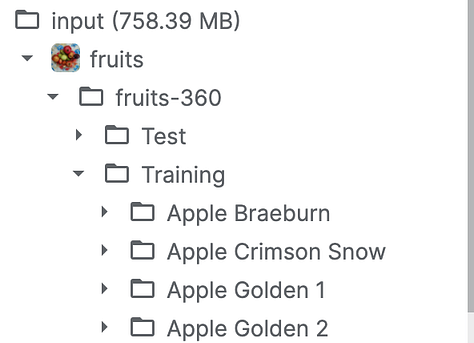Each type of fruits has its unique folder comprising of its jpg images.

Using matplotlib library to display the colour image:

``````import matplotlib.pyplot as plt

def show_example(img, label):
print('Label: ', dataset.classes[label], "("+str(label)+")")
plt.imshow(img.permute(1, 2, 0))
``````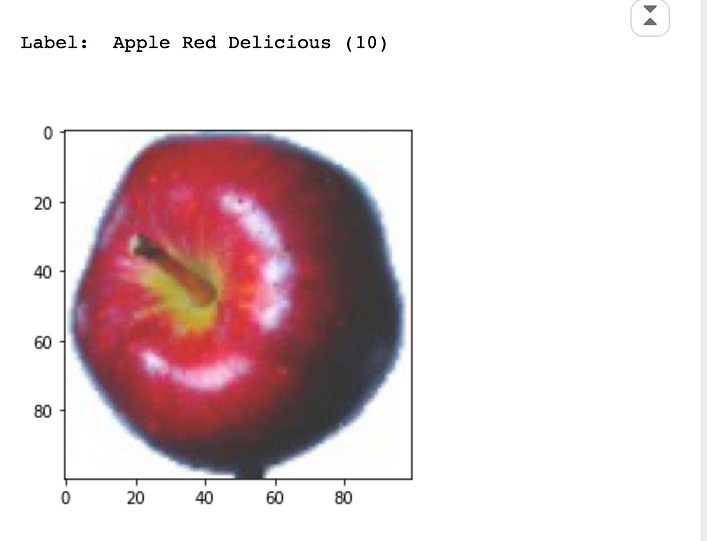dataset is an Apple Red Delicious

As we are using PyTorch, we need to convert the above pixel image into a tensor using `ToTensor`:

``````dataset = ImageFolder(data_dir + '/Training', transform=ToTensor())
img, label = dataset
print(img.shape, label)
img
``````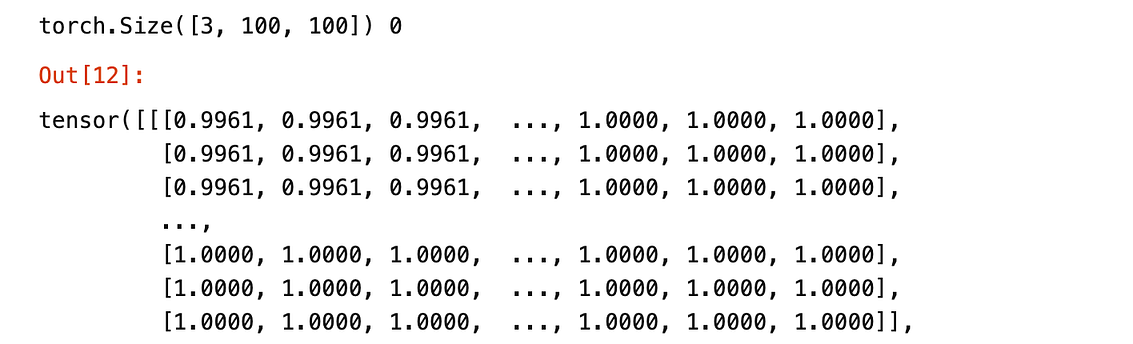An example of image converted into a tensor

There are 3 channels (red, green, blue) , 100*100 image size. Each value represents the colour intensity with respect to the channel colour.

Training and Validation Dataset

Next, we will randomly split the data to achieve 3 sets of data for different purposes.

1. Training Set : Train the model
2. Validation Set: Evaluate the model
3. Test Set: Report the final accuracy of the model

Size of training dataset : 57, 692

Size of validation dataset: 10, 000

Size of test dataset: 22, 688

Training in Batches

We have a total of 57, 692 training images. We should split our images into smaller batches before training our model using`_DataLoader_`. Working with a smaller set of data reduces memory space and in turn increases the speed of training.

For our dataset, we will use a batch size of 128.

![Image for post](https://miro.medium.com/max/1050/1*3Zp8qc3ZvGTzC7_r5ZFsWA.png

128 randomly assigned images in a training batch

Now, we will begin designing our models.

#neural-networks #pytorch #jovian #classification #resnet

## Buddha Community1595082060

## Classification of Fruit Images Using Neural Networks (PyTorch)

Objective: Find a neural network model that achieves the highest accuracy rate for the classification of Fruits360 images.

1. Deep Feed Forward
2. Convolutional Neural Network
3. Residual Neural Network (ResNet9)

Data Source: https://www.kaggle.com/moltean/fruits

Full Code Notebook: https://jovian.ml/limyingying2000/fruitsfinal

## Data Preparation

First, let’s understand our dataset!

The Kaggle Fruits 360 dataset consists of 90483 images of 131 different types of fruits and vegetables.

To begin, we import the data and the required libraries to run our codes.

``````import torch
import os
import jovian
import torchvision
import numpy as np
import matplotlib.pyplot as plt
import torch.nn as nn
import torchvision.models as models
import torch.nn.functional as F
from torchvision.datasets import ImageFolder
from torchvision.transforms import ToTensor
from torchvision.utils import make_grid
from torch.utils.data import random_split
import torchvision.models as models
%matplotlib inline
``````Each type of fruits has its unique folder comprising of its jpg images.

Using matplotlib library to display the colour image:

``````import matplotlib.pyplot as plt

def show_example(img, label):
print('Label: ', dataset.classes[label], "("+str(label)+")")
plt.imshow(img.permute(1, 2, 0))
``````dataset is an Apple Red Delicious

As we are using PyTorch, we need to convert the above pixel image into a tensor using `ToTensor`:

``````dataset = ImageFolder(data_dir + '/Training', transform=ToTensor())
img, label = dataset
print(img.shape, label)
img
``````An example of image converted into a tensor

There are 3 channels (red, green, blue) , 100*100 image size. Each value represents the colour intensity with respect to the channel colour.

Training and Validation Dataset

Next, we will randomly split the data to achieve 3 sets of data for different purposes.

1. Training Set : Train the model
2. Validation Set: Evaluate the model
3. Test Set: Report the final accuracy of the model

Size of training dataset : 57, 692

Size of validation dataset: 10, 000

Size of test dataset: 22, 688

Training in Batches

We have a total of 57, 692 training images. We should split our images into smaller batches before training our model using`_DataLoader_`. Working with a smaller set of data reduces memory space and in turn increases the speed of training.

For our dataset, we will use a batch size of 128.

![Image for post](https://miro.medium.com/max/1050/1*3Zp8qc3ZvGTzC7_r5ZFsWA.png

128 randomly assigned images in a training batch

Now, we will begin designing our models.

#neural-networks #pytorch #jovian #classification #resnet1597632480

## Build your own Neural Network for CIFAR-10 using PyTorch

In 6 simple steps

Neural network seems like a black box to many of us. What happens inside it, how does it happen, how to build your own neural network to classify the images in datasets like MNIST, CIFAR-10 etc. are the questions that keep popping up. Let’s try to understand a Neural Network in brief and jump towards building it for CIFAR-10 dataset. By the end of this article you will have answers to :

1. What are neural networks?
2. How to build a neural network model for cifar-10 dataset by using PyTorch?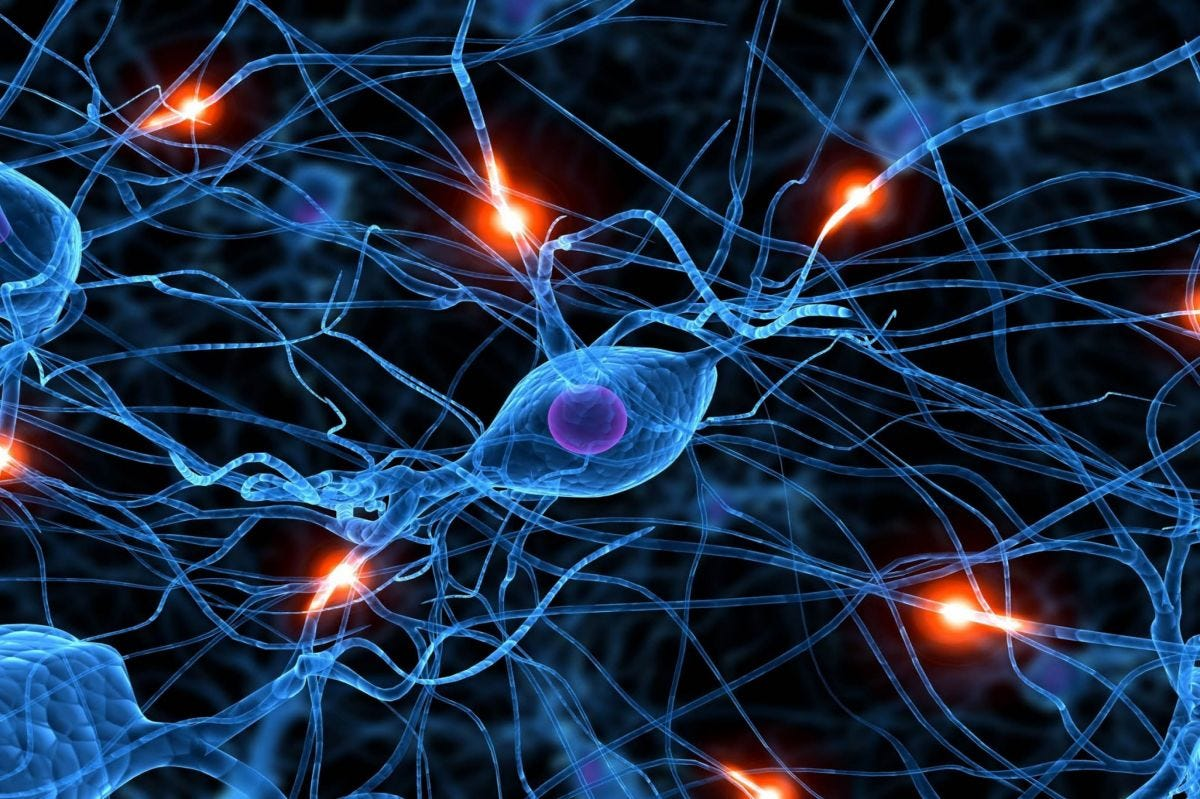### What are neural networks?

Neural networks(NN) are inspired by the human brain. A neuron in a human brain, individually is at rest until it collects signals from others through a structure called dendrites, when the excitation that it receives is sufficiently high, the neuron is fired up(gets activated) and it passes on the information. Artificial neural networks(ANN) are made up of interconnected model/artificial neurons(known as perceptron) that take many weighted inputs , add them up and pass it through a non-linearity to produce an output. Sounds simple!

#neural-networks #machine-learning #pytorch #cifar-10 #neural networks1597637160

## LeNet for Image Classification using GluonCV

In the previous article, we discussed about image classification using GluonCV on a pretrained network. In this article, we will discuss how to implement a binary image classifier by training LeNet by bringing different components of gluoncv together such as autograd, trainer, dataset, and dataloader, to train a LeNet network. We can accomplish this is by writing a training loop.

1. Import Libraries

We first import the libraries. We initialize mxnet.init for more parameter initialization methods, matplotlib for drawing, time for benchmarking as well as other gluon packages.

``````from mxnet import nd, gluon, init, autograd, metric
from mxnet.gluon import nn
from mxnet.gluon.data.vision import datasets, transforms

import matplotlib.pyplot as plt
from time import time
``````

2. Data

We will use **_fashion m-nest _**dataset for training.

Fashion m-nest dataset is automatically downloaded through gluonsdata.vision.datasets module. The dataset can be downloaded using the following code. It also shows the properties of the first example.

``````mnist_train = datasets.FashionMNIST(train=True)
x, y = mnist_train

print('X shape: %s dtype : %s' % (x.shape, x.dtype))
print('Number of images: %d'%len(mnist_train))
``````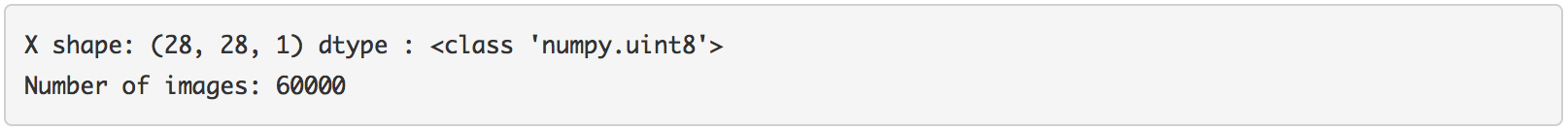Each example in this dataset is a 28 by 28 sides gray image which is presented as an NDRA with the shape format of height X width X channel. The label is a scalar.

#neural-networks #lenet-5 #deeplearing #gluoncv #image-classification #neural networks1596990660

## Image classification with PyTorch

CIFAR 10 Data set using logistic regression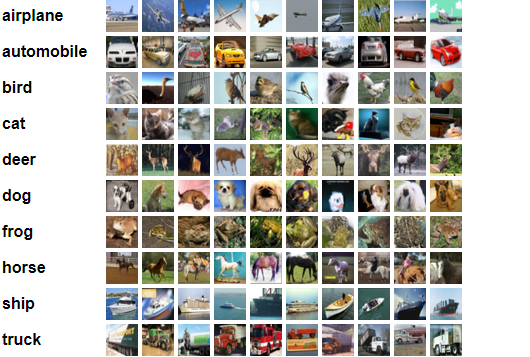In my previous posts we have gone through

Let us try to solve image classification of CIFAR-10 data set with Logistic regression.

Step 1 : Import necessary libraries & Explore the data set

We are importing the necessary libraries pandas , numpy , matplotlib ,torch ,torchvision. With basic EDA we could infer that CIFAR-10 data set contains 10 classes of image, with training data set size of 50000 images , test data set size of 10000.Each image is of [3 x 32 x 32 ]. Which represents 3 channels RGB,32 x 32 pixel size.

``````#Explore CIFAR Data set
test_dataset = CIFAR10(root='data/', train=False, transform=ToTensor())
#size of training data
dataset_size = len(dataset)
dataset_size
#size of test data
test_dataset_size = len(test_dataset)
test_dataset_size
#number of classes in the data set
classes = dataset.classes
classes
``````

Visualizing a sample image and the size of the sample image.

``````#Let us understand the size of one image
img, label = dataset
img_shape = img.shape
img_shape
#Let us look at a sample image
img, label = dataset
plt.imshow(img.permute((1, 2, 0)))
print('Label (numeric):', label)
print('Label (textual):', classes[label])
``````

As this is a 3 channel RGB image Pytorch expects the channels as first dimension where as matplotlib expects as last dimension of the image.Here .permute tesnor method is used to shift channels to last dimesnion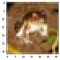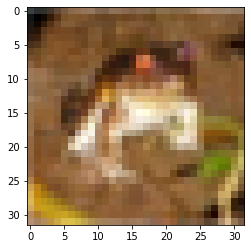Label (numeric): 6

Label (textual): frog

Step 2 : Prepare data for training

We using training set , validation set , Test set. Why we need them ?

Training set : used to train our model,computing loss & adjust weights Validation set : To evaluate the model with hyper parameters & pick the best model during training. we are using 10% of training data as validation set Test data set : Used to compare different models & report the final accuracy.

We are using the random_split from pytorch for creating train_ds,val_ds. torch.manual_seed(43) is set for reproducing the results.

``````#validation set size 5000 ie 10%
torch.manual_seed(43)
val_size = 5000
train_size = len(dataset) - val_size
#creating training & validation set using random_split
train_ds, val_ds = random_split(dataset, [train_size, val_size])
len(train_ds), len(val_ds)
``````

We are using the data loader as we used in our previous example , with a batch size of 128. To visualize our data we are using make_grid helper function from torch vision.

#pytorch #neural-networks #image-classification #deep-learning #logistic-regression #deep learning1598554500

## Satellite image classification with a convolutional neural network.

My latest project at Flatiron was to use neural networks to classify satellite image tiles. I chose to use a convolutional neural network (CNN) and create a dataset of webscraped images to train the model with. This will just be a quick rundown of what went into the project with additional links to my articles to more of the technical parts. This way, it can help to familiarize you with the topics or help to share more about my work with those who have similar interests in computer vision and machine learning.

I chose to use a CNN because I read some school lessons on computer vision about how a CNN has advantages with image classification. A CNN uses pooling layers that filter through patches of the image pixels, finding common patterns, which develop into more complex patterns in order to help determine image class. I chose to work on a computer vision project with satellite images because there are possible use cases for solutions on Earth as well as use cases on other planets. I’ve read articles about organizations looking at different geological patterns on the Mars surface in search of the possible presence of water or perhaps its pre-existence on the planet. This led me to try and build the model to recognize river delta patterns here on Earth, with the next step being to train the model and locate delta patterns on Mars. The model could also eventually be useful for looking at changes to river deltas on Earth, for possible use in agriculture, climate change or even real estate. For now, the project is ongoing as of my writing this blog post, with training and testing performed on the Earth images. The Mars images will be the next part I’ll begin after graduation.

A land image tile.

A river delta image tile.

A Mars delta image tile.

In order to obtain the images for a dataset, I looked into some different API’s and webscraping with Beautiful Soup. Afterwards, I decided to use Selenium to scrape some images from an image search on Google. This method was able to scroll through the page interactively, which was necessary in order to have access to all of the images. I wrote a separate article about that process here. This method was useful as a starting point, in order to go through the process of building the dataset, creating the model, training, testing and just getting everything to work. The disadvantage was that there were a lot of images that were not clean, contained pieces of text or other image artifacts and overall led to less accurate results. There are some example images below so you can see what I mean. I do not claim copyright to any of the used images as they were used for an educational project for school and will remove them if anyone objects to their display on my article.

#convolutional-network #computer-vision #python #image-classification #machine-learning #neural networks